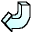Back### Solution 3

3x - 2 = x + 10

Subtract x from both sides

3x - x - 2 = x - x + 10

2x - 2 = 10

Add 2 to both sides

2x - 2 + 2 = 10 + 2

2x = 12

Divide both sides by 2

x = 6

### Check!

3 x 6 - 2 = 16 = 6 + 10